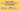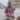# How to Use Array Reduce Method in JavaScript

Posted April 13, 2021Start discussion

Let’s say you have an array of numbers:

`javascript`const numbers = [2, 4, 6];``

How can you sum these numbers? Thanks to the `array.reduce()` method of JavaScript, you can easily do that:

`javascript`const numbers = [2, 4, 6];const sum = numbers.reduce(function(sum, number) {  const updatedSum = sum + number;  return updatedSum;}, 0);sum; // 12``

In this post, you’ll learn about the reduce operation, and how to use `array.reduce(callback, initialValue)` method to perform the reduce operation on arrays.

## 1. Reduce operation

Before diving into how to use the `array.reduce()` method, let’s find distinguish what problem it tries to solve: reducing an array to a value.

Reducing an array meaning performing a concrete accumulative operation on its items to calculate a result value.

A good example of reduce operation is calculating the sum of an array, where the accumulative operation is the addition of items. For an array `[2, 4, 6]` reducing to a sum results in `12`.

Another example of reducing is determining the maximum element of the array. For an array `[7, 2, 10, 6]` the reducing to max item results in `10`.

Now you can easily see what` array.reduce()` method does — perform a reduce operation.

## 2. array.reduce() method

The `array.reduce()` is a method on array that accepts 2 arguments:

`javascript`const value = array.reduce(callback[, initialValue]);``

The `callback` is an obligatory argument that is a function performing the reduce operation, and the second optional argument is the initial value.

JavaScript invokes the `callback` function upon each item of the array with 4 arguments: the accumulator value, the current array item, the current array item index, and the array itself. The `callback` function must return the updated accumulator value.

`javascript`array.reduce(function(accumulator, item, index, array) {  // Use `accumulator` and `item`   // to calculate `updatedAccumulator`...  return updatedAccumulator;})``

For example, let’s get back to the code from the introduction:

`javascript`const numbers = [2, 4, 6];const sum = numbers.reduce(function summarize(sum, number) {  const updatedSum = sum + number;  return updatedSum;}, 0);sum; // 12``

Try the demo.

`numbers.reduce(summarize, 0)` calculates the sum of all elements in the array.

The `summarize` callback is invoked for every item in the array with the accumulated sum and the iterated number. `summarize` callback adds the iterated item to the already accumulated sum, and returns that updated sum.

That’s how an array is reducing to a sum.

Also, note the second argument of `numbers.reduce(summarize, 0)` — the sum of array items is initialized with `0`.

Side challenge: how would you use `array.reduce()` method to find the maximum value of the array? Write your solution in a comment below!

## 3. Omitting initial value argument

You can also omit the second argument of the `array.reduce()`. In such a case the reduce method initializes the accumulator value with the first item of the array, and the iteration starts from the second item.

For example, let’s sum the array items without indicating the second argument of `number.reduce(summarize)`:

`javascript`const numbers = [2, 4, 6];const sum = numbers.reduce(function summarize(sum, number, index) {  console.log(index); // logs 1, 2  return sum + number;});sum; // 12``

Try the demo.

The initial value argument is omitted, thus the accumulator is initialized with the value of the first item (the number `2`). The iteration starts from the second item.

## 4. Conclusion

`array.reduce()` is a useful method that reduces an array to a value.

`array.reduce(callback, initialValue)` accepts 2 arguments: the `callback` function that updates the accumulator value, and the initial value of the accumulator. `array.reduce()` then invokes the `callback` for each item of the array, updating the accumulator at each step.

You can also omit the initial value argument, in which case the accumulator value is initialized with the first item of the array, and the iteration starts from the second item.

Would you like to read more about array methods? Follow my post on How to Use forEach() to Iterate an Array.

## Quality posts into your inbox

I regularly publish posts containing:

• Important JavaScript concepts explained in simple words
• Overview of new JavaScript features
• How to use TypeScript and typing
• Software design and good coding practices